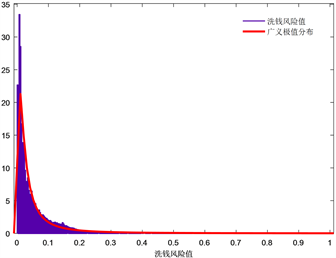﻿ 基于银行流水数据的洗钱风险综合评估

# 基于银行流水数据的洗钱风险综合评估Comprehensive Evaluation of Money Laundering Risk Based on Bank Flow Data

Abstract: According to the behavior characteristics of money-laundering, the comprehensive evaluation index system of money-laundering risk is established on the basis of the flow data of bank personal customers. The method of factor analysis and entropy method are used to establish the comprehensive evaluation model of money-laundering risk, and the generalized extreme value (GEV) distribution is used to fit the distribution of comprehensive risk value of money-laundering. The empirical analysis is carried out by using the customers personal flow data of a commercial bank in Chongqing in 2018. The results show that the risk value of money-laundering of most bank individual customers is relatively low, and only a few customers may have money-laundering behavior. According to the fitted comprehensive risk distribution, at the significant level of 0.05, there are 5239 (2.66%) customers who may have money-laundering risk, and they need further identification, which will undoubtedly greatly improve the efficiency of the bank.

1. 引言

2. 银行个人客户洗钱风险综合评价模型

2.1. 个人客户流水数据采集

2.2. 洗钱风险综合评价指标体系

2.2.1. 洗钱风险评价指标的选取原则

① 科学性原则。洗钱风险评估的各项指标应建立在科学实用的基础上，要有清晰明了的层次结构，并且能真实客观地反映银行客户的交易情况。

② 全面性原则。选取的评价指标不仅需要全面反映出与客户洗钱相关的各方面信息，还能够相互配合。

③ 可操作性原则。评价指标所需数据必须准确，易于采集。

④ 公正性原则。选取的洗钱风险评价指标必须客观反映出客户的交易情况，不能偏袒。

⑤ 可比性原则。评价指标的选取要具有概括性和普遍性，便于能够准确科学地进行纵向或横向比较。

2.2.2. 洗钱风险综合评价指标体系Table 1. Integrated evaluation index system for money laundering risk

2.3. 洗钱风险综合评价模型

2.3.1. 综合评价指标的降维优化Table 2. Factor total variance explanation

2.3.2. 因子旋转与公因子命名Table 3. Factor load matrix after rotationTable 4. Common factor composition of risk assessment index system for money laundering

2.3.3. 基于熵值法的洗钱风险综合评估模型

① 对第j项指标进行等分分段，统计每段范围内所包含的客户个数，并计算每段范围的概率： ${P}_{ij}={X}_{ij}/{\sum }_{i=1}^{k}{X}_{ij}，\text{\hspace{0.17em}}i=1,2,\cdots ,k;\text{\hspace{0.17em}}j=1,2,\cdots ,m$，这里的表示将第j项指标等分为k段， ${X}_{ij}$ 表示第j项指标第i段范围内所包含的客户个数。

② 计算第j项指标的熵值： ${e}_{j}=-a×\underset{i=1}{\overset{k}{\sum }}{P}_{ij}\mathrm{ln}\left({P}_{ij}\right)$，其中 $a=1/\mathrm{ln}\left(k\right)>0$$0\le {e}_{j}\le 1$

③ 计算第j项指标的差异系数： ${g}_{j}=1-{e}_{j}$

④ 求第j项指标的权重： ${w}_{j}={g}_{j}/{\sum }_{j=1}^{m}{g}_{j}$Table 5. Entropy value and weight of evaluation index

${F}_{i}=\underset{j=1}{\overset{6}{\sum }}{{F}^{\prime }}_{ij}×{w}_{j}\text{\hspace{0.17em}}\text{\hspace{0.17em}}\left(i=1,\text{\hspace{0.17em}}2,\text{\hspace{0.17em}}\cdots ,\text{\hspace{0.17em}}n\right)$ (1)

3. 实证研究

3.1. 重庆市某商业银行个人客户洗钱综合风险计算Table 6. Comprehensive evaluation of money laundering risk for top ten customers

3.2. 洗钱风险分布拟合

$F\left(x\right)=\mathrm{exp}\left\{-{\left[1+k\left(\frac{x-\mu }{\sigma }\right)\right]}^{-\frac{1}{k}}\right\}$ (2)Figure 1. Matched chart of integrated value density function of money laundering risk

$\text{\hspace{0.17em}}F\left(x\right)=\mathrm{exp}\left\{-{\left[1+0.86\left(\frac{x-0.018}{0.022}\right)\right]}^{-\frac{1}{0.86}}\right\}$ (3)

3.3. 银行个人客户洗钱风险总体评估Table 7. Possibility of money laundering at different significant levels and number of clients

4. 结论

 陈云开, 卢正鼎, 刘芳, 等. 一种高维聚类算法及在洗钱侦测中的应用[J]. 计算机科学, 2007(6): 195-197+217.

 丁佳, 封思贤. 第三方支付机构洗钱风险的评估方法研究[J]. 金融发展研究, 2018, 444(12): 51-58.

 丁昱. 商业银行个人客户洗钱静态风险分类方法研究[J]. 金融理论与实践, 2017(8): 59-63.

 肖琨, 王云, 张桂刚. 基于识别和多重分类的反洗钱系统[J]. 小型微型计算机系统, 2019(10): 2046-2051.

 金大卫. 我国反洗钱监控机制的完善及创新[J]. 经济管理, 2010(4): 28-34.

 童文俊. 金融机构洗钱风险评估监管体系构建研究[J]. 金融与经济, 2013(2): 73-76.

 王宝运, 王怡靓. 我国商业银行反洗钱有效性影响因素分析[J]. 金融理论与实践, 2017(7): 99-103.

 胡爱平, 邱世芳, 伍度志. 重庆市城镇化综合评价指标体系应用研究[J]. 重庆理工大学学报(自然科学), 2016, 30(03): 140-148.

Top